## The Tiger Algebra Solver App allows you to easily get answers and solutions for Algebra Problems in A variety of topics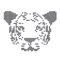# Tiger Algebra Solver

by 7berge GmbH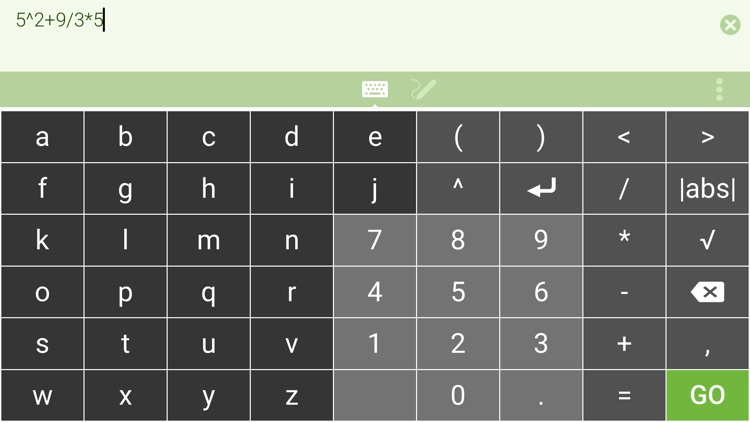The Tiger Algebra Solver App allows you to easily get answers and solutions for Algebra Problems in A variety of topics. The Algebra Solver, Simplifier and Evaluator currently handles :### App Details

Version
3.0.2
Rating
(80)
Size
26Mb
Genre
Education
Last updated
October 17, 2019
Release date
September 11, 2014

• \$3.99

### App Screenshots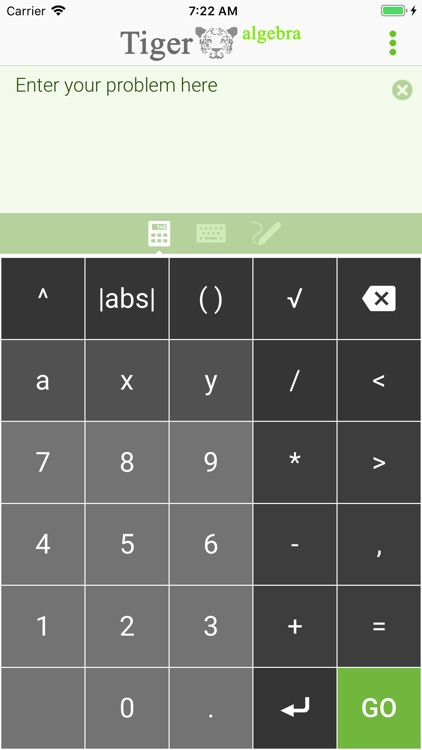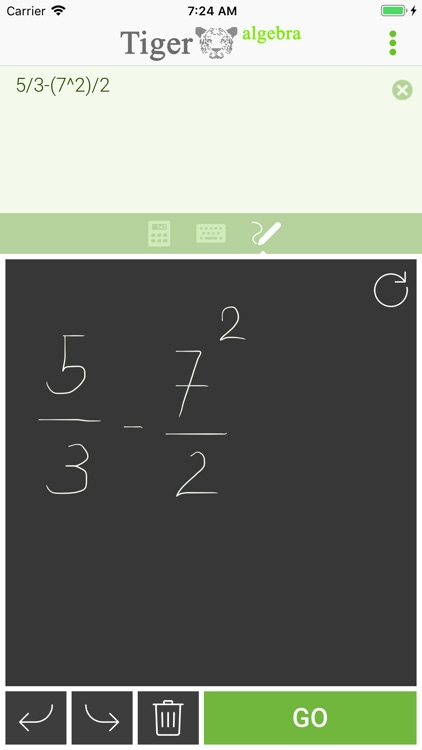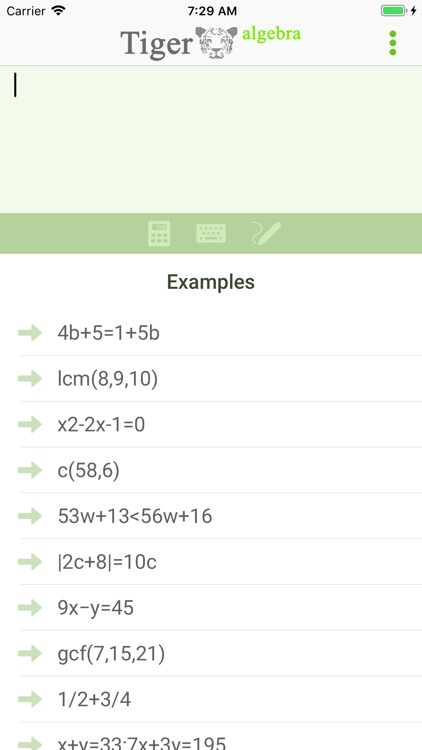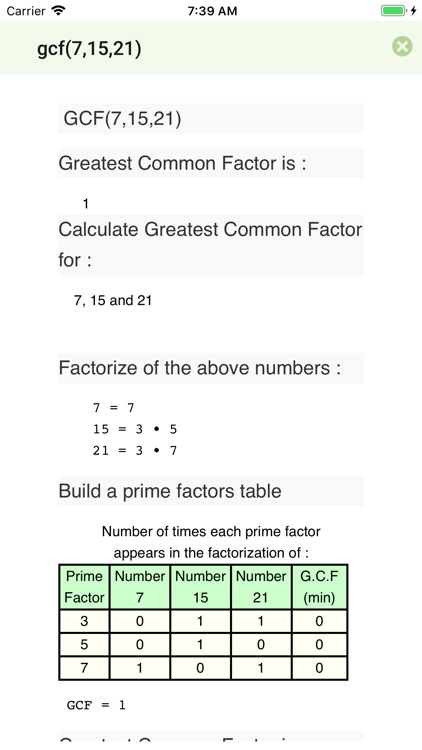### App Store Description

The Tiger Algebra Solver App allows you to easily get answers and solutions for Algebra Problems in A variety of topics. The Algebra Solver, Simplifier and Evaluator currently handles :

Pulling Out Like Terms
Identifying Perfect Cubes
Canceling out
Reducing Fractions to Lowest Terms

Solving by Completing The Square
Equations which are Reducible to Quadratic
Parabola Finding Vertex and X Intercepts
Fractions

Adding Subtracting Finding Least Common Multiple
Multiplying Fractions
Dividing Fractions
Exponents

Raising to a Power
Dividing Exponents
Multiplying Exponents
Sets of Linear Equations

Linear Equations with One Unknown
Linear Equations with Two Unknowns
Linear Equations with Three Unknowns
Linear Equations with Four Unknowns
Solving Linear Equations by Substitution
Properties of a Straight Line
Non Linear Equations and Approximation and Inequalities

Non Linear Equations
Approximation
de Moivre Formula
Linear Inequalities
Factorization

Factoring Multi Variable Polynomials
Factoring Binomials as Difference of Squares
Factoring Binomials as Sum or Difference of Cubes
Factoring Trinomials
Factoring Polynomials having more than 3 Terms
Other Factorizations
Polynomial Root Calculator
Polynomial Long Division
Absolute Value

Absolute Value Equations
Absolute Value Inequalities
Progressions

Arithmetic Series
Geometric Series
Geometry

Distance Between Two Points And Their Midpoint
Find Area of Triangle given by its 3 sides
Calculators

GCF Greatest Common Factor
LCM Least Common Multiple
Find Prime Factors
Basic Complex Operations
Square Root Simplifier

http://www.tiger-algebra.com/

Disclaimer:
AppAdvice does not own this application and only provides images and links contained in the iTunes Search API, to help our users find the best apps to download. If you are the developer of this app and would like your information removed, please send a request to [email protected] and your information will be removed.